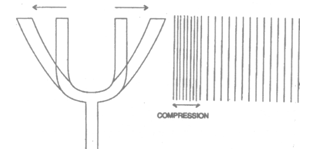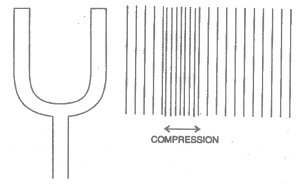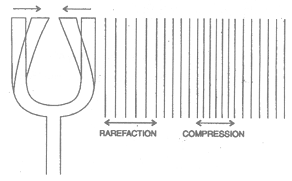# PROPAGATION OF SOUND

## Sound of Class 9

From the given two experiments we know that sound is propagating from bell B to pitch ball P.But the question is how the sound is propagating to understand this  lets do a experiment take a tuning fork that fork is a source of standard frequency for this experiment. Initially it is set into vibrations and its prongs A and B are kept vertical. The prongs can move in and out from their means position and have a transverse vibratory motion. When the prongs are in means position, the air in their surrounding has normal density. That means no disturbance in surrounding. That you can understand by given figure.when we strike the tuning fork it vibrates as the right prong moves out towards right, it pushes the air layers to the right. This produces a compression in air that shown in the figure with closer  lines.In the given figure we can understand this disturbance in surrounding by tuning fork.After some moment of time the prong returns inwardly to its mean position. The compression in the air moves towards the right. But the air near the prong again has normal density as shown in figure.It means vibration in the air is propogate towards right when the tuning fork is striking the air in right.As the prong continues moving toward s extreme left, vacating the space, density of air falls in the region and a rarefaction is produced (It is shown in figure (d) with spread lines).

As the prong moves back to right extreme, it competes one vibration. Also the motion of the prong produces a new compression. This completes one wave.

Since on vibration of the prong has generated one wave in the medium (air), in one second and many waves will be generated as the number of vibrations that the tuning fork will make in one second. This number is called frequency of the tuning fork (This number is engraved on the tuning fork near the bend). Hence we conclude that the wave frequency (the number of waves being generated per second) is equal to the frequency of the tuning fork.